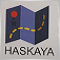35

Greetings earthlings, how can I close a partial order such as 1/2 of my total position after it reaches 1 ATR profit?  once the below code triggers it keeps removing half of the position that is left. I was thinking of using my lotsize from the order but i have it as a function that returns the lot value so i couldn't store it as a variable and compare that to the current orderlots. How can I get around this? The help is much appreciated!

```for(int i = OrdersTotal()-1;i>=0;i--)
{
{
if(OrderType() == OP_SELL && OrderMagicNumber() == MN && ChartSymbol() == Symbol())
{
if (Close <= OrderTakeProfit()+ATR)
{

if (res != true)
{
int err = GetLastError(); Alert("LastError = ",err);
}

}
}
else if (OrderType() == OP_BUY && OrderMagicNumber() == MN && ChartSymbol() == Symbol())
{
if(Close >= OrderTakeProfit()-ATR)
{

if (res != true)
{
int err = GetLastError(); Alert("LastError = ",err);
}
}```23747

1. You can't just use OrderLots()/2 because that is not a multiple of LotStep, and you can't close or have remaining less than MinLot.

2. You also must check if you have already done it, to avoid repeated closing. Alternatives:

• Move SL to Break Even+1 before the partial close. That way you know that you already did it.

• Set a flag in persistent storage (files, global variables w/flush)

• Open two orders initially, and close one (manually or by TP.)2215

nicholas herrera:

Greetings earthlings, how can I close a partial order such as 1/2 of my total position after it reaches 1 ATR profit?  once the below code triggers it keeps removing half of the position that is left. I was thinking of using my lotsize from the order but i have it as a function that returns the lot value so i couldn't store it as a variable and compare that to the current orderlots. How can I get around this? The help is much appreciated!

```for(int i = OrdersTotal()-1;i>=0;i--)
{
{
if(OrderType() == OP_SELL && OrderMagicNumber() == MN && OrderSymbol() == Symbol())
{
if (Close <= OrderTakeProfit()+ATR)
{
bool res = OrderClose( OrderTicket(), NormalizeDouble((OrderLots()/2),2), MarketInfo(OrderSymbol(), MODE_ASK), 5, CLR_NONE );

if (res != true)
{
int err = GetLastError(); Alert("LastError = ",err);
}

}
}
else if (OrderType() == OP_BUY && OrderMagicNumber() == MN && OrderSymbol() == Symbol())
{
if(Close >= OrderTakeProfit()-ATR)
{
bool res = OrderClose( OrderTicket(), NormalizeDouble((OrderLots()/2),2), MarketInfo(OrderSymbol(), MODE_BID), 5, CLR_NONE );

if (res != true)
{
int err = GetLastError(); Alert("LastError = ",err);
}
}
```